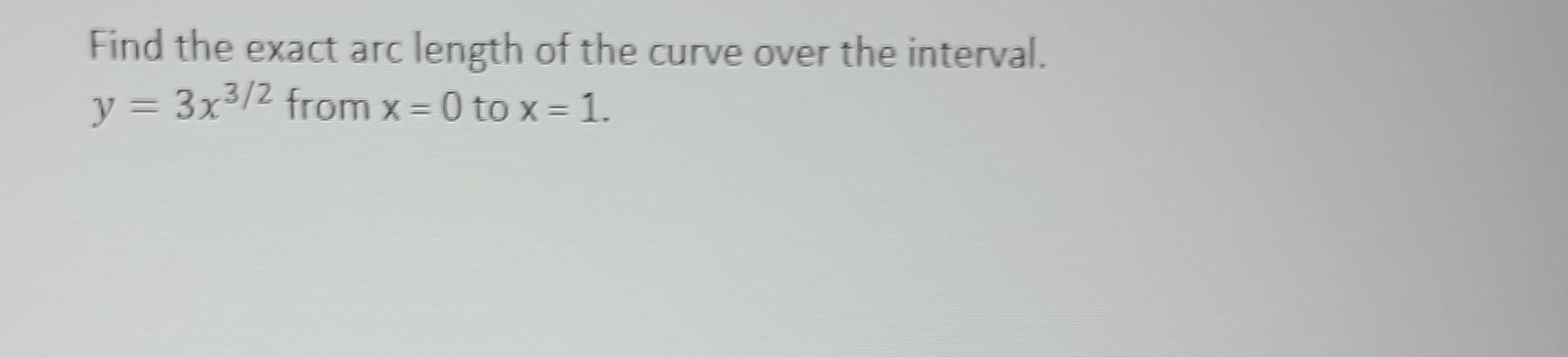Home / Expert Answers / Calculus / nbsp-find-the-exact-arc-length-of-the-curve-over-the-interval-y-3-x-3-2-from-x-0-pa384

# (Solved):   Find the exact arc length of the curve over the interval. \( y=3 x^{3 / 2} \) from \( x=0 ...Find the exact arc length of the curve over the interval. \( y=3 x^{3 / 2} \) from \( x=0 \) to \( x=1 . \)

We have an Answer from Expert